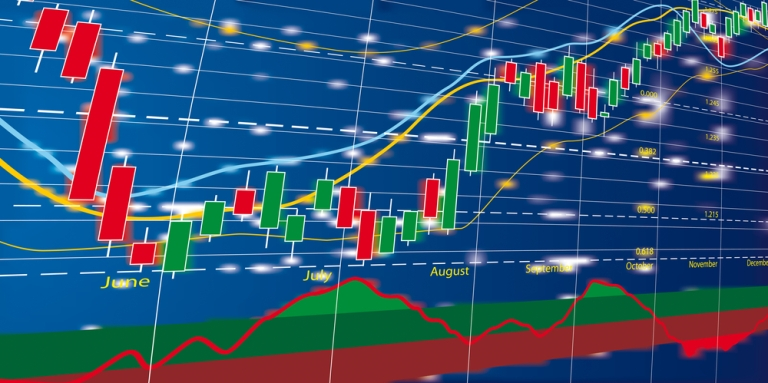# Cracking Ratios – Ratio Analysis

No comment 254 views
5 / 5 ( 1votes )Ratio Analysis

Ratio Analysis is the most commonly used analysis to judge the financial strength of a company. A lot of entities like research houses, investment bankers, financial institutions and investors make use of this analysis to judge the financial strength of any company.

This analysis makes use of certain ratios to achieve the above-mentioned purpose. There are certain benchmarks fixed for each ratio and the actual ones are compared with these benchmarks to judge as to how sound the company is. The ratios are divided into various categories, which are mentioned below:

Profitability ratios

Profitability ratios speak about the profitability of the company. The various profitability ratios used in the analysis are, operating margin (operating profit divided by net sales), gross margin (gross profit divided by net sales) net profit margin (net profit divided by net sales), return on equity (net profit divided by net worth of the company) and return on investment (operating profit divided by total assets). As obvious from the name, the higher these ratios the better for the company.

Solvency ratios

These ratios are used to judge the long-term solvency of a firm. The most commonly used ratios are – Debt Equity ratio (total debt divided by total equity), Long term debt to equity ratio (long term debt divided by equity). While the accepted norm for debt equity ratio differs from industry to industry, the usual accepted norm for D/E is 2:1. It should not be more than this. For certain industries, a higher D/E is accepted, e.g., in banking industry, a debt equity ratio of 12:1 is acceptable.

Liquidity ratios

These ratios are used to judge the short-term solvency of a firm. These ratios give an indication as to how liquid a firm is. The most commonly used ratios are – Current ratio (all current assets divided by current liabilities) and quick ratio (current assets except inventory divided by current liabilities). The accepted norm for current ratio is 1.5:1. It should not be less than this.

Turnover ratios

These ratios give an indication as to how efficiently a company is utilizing its assets. The most commonly ratios are sales turnover ratio, inventory turnover ratio (average inventory divided by net sales) and asset turnover ratio (net sales divided by total assets). The higher these ratios, the better for the company.

Valuation Ratios

Valuation ratios give an indication as to whether the stock is underpriced or overpriced at any point of time. The most commonly used ratios are Price to Earnings (P/E) ratio and price to book value (PBV) ratio. But care has to be taken while interpreting these ratios. While P/E ratio of a company should be compared with the industry P/E and the P/E of the competitors, it is the PBV that can distort.

While a lower PBV usually means a lower valuation, there can be a case where a low PBV can be because of a very huge capital base of the company. In such a case, the stock might be overvalued but the PBV will indicate that the stock is undervalued. On the other extreme, a higher PBV usually means overvalued stock but that can also be because the company has a very small capital base. So care has to taken while interpreting these ratios.

Coverage ratios

These ratios give an indication about the repayment capabilities of a company. The most commonly used coverage ratios are Interest coverage ratio (Interest outstanding divided by earnings before interest and taxes) and debt service coverage ratio (earnings before interest and taxes plus all non cash charges divided by interest outstanding plus the term loan repayment installment). The acceptable norm for DSCR is 2:1.

Tags:Forex4Live.com is a forex trading system website that started to be published since 2011 and has been used by thousands of traders around the world. The system is very simple and accurate, priced at \$ 167 for a lifetime membership with no monthly fees or hidden fees. Our team created this system by testing thousands of indicators then selecting 23 indicators and combining them into one great trading system.
No Response